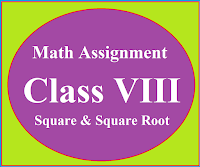## Posts

Showing posts from March, 2019

### Math Assignment Class VIII | Square & Square RootMath Assignment  Class VIII | Square & Square Root Download or Print free  assignment with answer key  for   Class  8 Squares and  Square Roots.   Important and extra questions that cover all topics of square and square root and is useful and helpful for the students. Math Assignment  Class VIII | Square & Square Root LEVEL -1HOME PAGE FOR THE SITE CBSEMATHEMATICS.COM CBSE mathematics  for 7th, 8th, 9th, 10th 11th and 12th classes. Maths formulas for the students, Step by step maths basic concepts for the students. Maths practical and project work for the students.   Reading and teaching mathematics is a challenge for the people. The main reason here is that most of the people read mathematics but they don't have interest in it. Without interest students cannot read mathematics for longer time so they decided to quit. If a student study mathematics step by step then surely the subject become interested for him. Through this blog we will try our best to reach the needs of the students and make mathematics interesting and valuable to them MATHEMATICS RESOURCE CENTRE   Mathematics resource centre contains chapter-wise mathematics formulas worksheets, assignments, lesson plans, MCQ and case studies on mathematics and many other important links.   Mathematics Formulas and Assignments By clicking on the lin

### Class X Maths Formulas | Basics | Assignments | SPCBSE Class 10th Maths Assignments and Sample Papers CBSE syllabus, sample paper, unit test and assignments for mathematics for class IX and X. Chapter Wise  assignments for classes IX & X  for mathematics. Chapter-wise basic concepts & formulas for classes IX & X, Assignment pdf for math for classes IX & X ********************************** Case Study Based Questions Click Here   Maths Practical (Classes IX to XII) For 2022-23 Click Here   Maths Sample Paper (Class IX to XII) For 2022-23 Click Here RESOURCE CENTRE MATHEMATICS ************************************ TEACHER'S NOTES SUBJECT:  MATHEMATICS CLASS 10 CLASS 10 (Basic and Standard Mathematics) Chapter 1 Chapter 2 Chapter 3 Chapter 4 Chapter 5 Chapter 6 Chapter 7 Chapter 8 Chapter 9 Chapter 10 Chapter 11 Chapter 12 Chapter 13 Chapter 14 Chapter 15 *****************************   Class X NEW NCERT BOOK OF MATHEMATICS SESSION (2023-24)  Mind Map in Mathematics : Click Here